# Catch up on the latest AI articles# [PETRv2] Estimates The 3D Position Of An Object Using Only Camera Images.

3 main points
✔️ A method for 3D object recognition using only camera images, which has attracted particular attention in the area of automated driving
✔️ In addition to object recognition,
it also performs segmentation of bird's-eye view images and 3D lane recognition simultaneously
✔️ A transformer-based method that adds 3D information + time series information to position embedding

written by Yingfei LiuJunjie YanFan JiaShuailin LiAqi GaoTiancai WangXiangyu ZhangJian Sun
(Submitted on 2 Jun 2022 (v1), last revised 14 Nov 2022 (this version, v3))

Subjects: Computer Vision and Pattern Recognition (cs.CV)code：The images used in this article are from the paper, the introductory slides, or were created based on them.

## Introduction

PETR v2, as the name suggests, is an extension of PETR (Position Embedding TRansformer), which solves the task of estimating the 3D position of an object using only images from multiple cameras, a task that has attracted much attention in the area of automated driving. PETR is a method for solving the task of estimating the 3D position of an object using only images from multiple cameras. PETR v2 takes into account time-series information by using images from multiple cameras for multiple time frames PETR v2 has been extended to take into account time-series information by using images from multiple cameras for multiple time frames. In addition to 3D object recognition, PETR v2 can also perform segmentation of bird's-eye view images (classifying each pixel in the bird's-eye view into driveable areas, lanes, and cars) and 3D lane detection at the same time.

In this article, I will first give an overview of PETR, followed by an overview of PETR v2 and details of the changes from PETR.

## PETR (Position Embedding TRansformer)

PETR is a transformer-based 3D object detection method. First, features are calculated from images acquired by multiple cameras using a common backbone network such as ResNet-50, and then 3D features are created by adding 3D positional embeddings calculated from the camera positional relationships to the features. The values are then transformed by Transformer. The values are then used as the Transformer's key and value, and are entered into the Transformer together with the object query calculated from the 3D points in space to update the query, and the class label and BBox parameters are calculated for each updated query. The class label and BBox parameters are calculated for each updated query.

Next, we will explain thecalculation of 3D position embedding, query updating by the Transformer, and the loss calculationpart, respectively.

### 1. calculation of 3D position embedding

Assume that the dimension of the features computed from each image is C x Hf x Wf.

First, from the camera FOV space called Camera Frustum Space, consider D x Hf x Wf grid points as shown in the figure below, where D represents the number of grid points in the depth direction.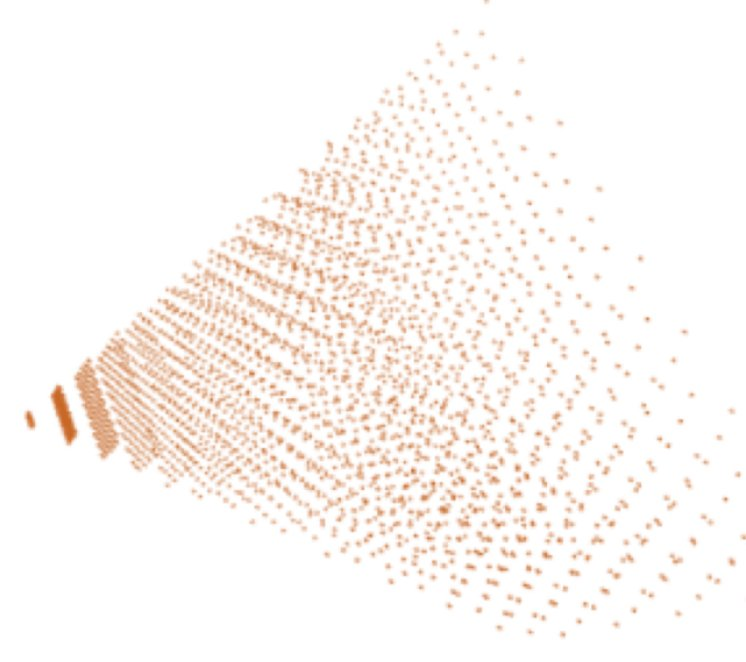These grid points are transformed from the camera coordinate system to the world coordinate system using a matrix calculated from each camera's orientation. By putting these points into MLP, a 3D position embedding is obtained. Then, by applying 1x1 conv to the image features to change their dimensions and adding the 3D position embedding to the image features, we can obtain image features that take the 3D position into account.

### 2. update of object query by Transformer

First, we explain how the object query input to the Transformer is computed: PETR first sets up several random anchor points (trainable parameters) in the 3D space. The number of anchor points is the maximum number of objects that can be detected. The object query is calculated by putting the coordinates of each of these points into MLP.

Then, by inputting this value as query and the image features that take into account the 3D position calculated earlier as key and value into the Transformer Decoder, the value of each query is updated.

### 3. loss calculation

The query obtained from the Transformer is input to the class label estimation and BoundingBox estimation networks, respectively. For the class label estimation results, the focal loss is used, and for the BBox, the loss is calculated using the Hungarian algorithm by estimating the offset from theanchor point from which the object query was created.

Loss calculation using the Hungarian algorithmis also used in DETR, a 2D object detection method utilizing Transformer, and DETR3D, a 3D version of it. Unlike conventional object detection methods that use anchor boxes, it is not known which of the actual objects (=Ground Truth) corresponds to the BBox generated from a certain query, so a cost function is calculated for each combination of the query and the actual object, The optimal combination is calculated by the Hungarian algorithm. Once the best combination is found, the loss of the parameters of BBox is calculated using L1 loss as usual.

The above is an overview of PETR.

## PETR v2

The figure below shows the process flow of PETR v2. the major changes from PETR are: 1) it now uses not only observations at the current time as input but also past observations, and 2) it now performs not only object detection but also segmentation and lane estimation on a bird's-eye view.
C is the Concat process, and A stands for Align, which refers to the process of converting a 3D position embedding calculated in a coordinate system based on the vehicle position or LiDAR position at the previous time into a coordinate system based on the current position.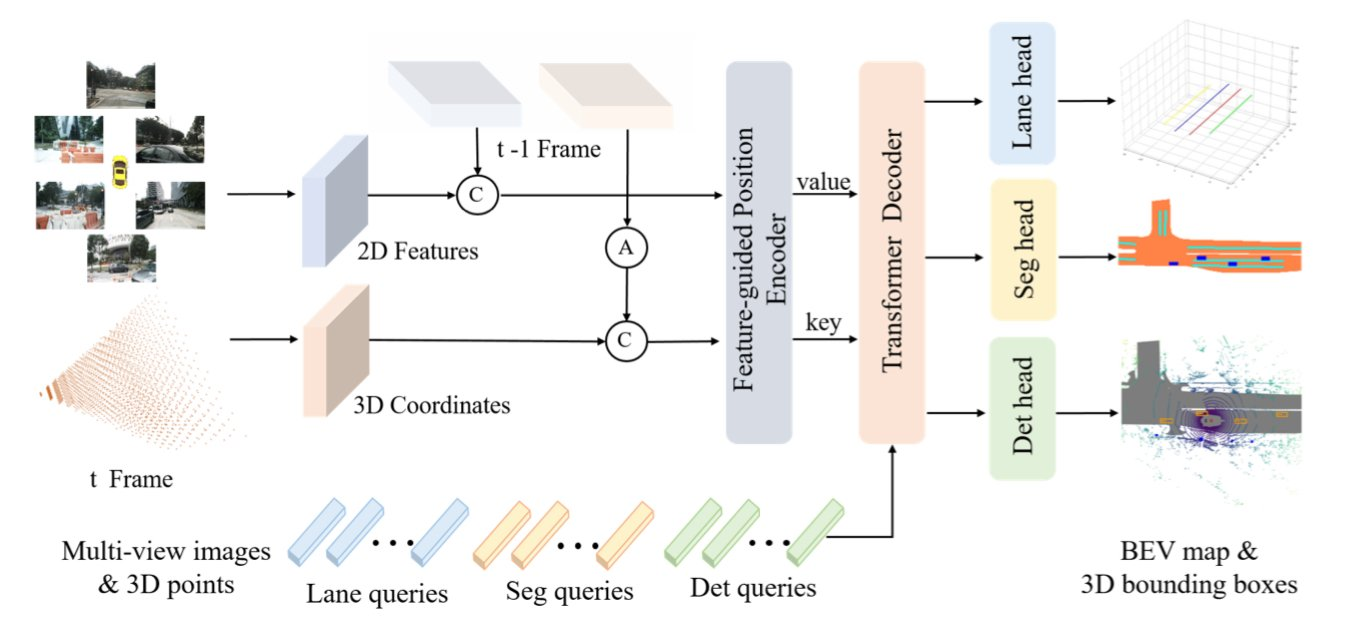We will now go on to explain the details of the changes from PETR one by one.

### 1. 3D position embedding calculation utilizing time series data

As in PETR, the 3D position embedding is calculated by transforming the grid points in the camera's FOV space, called Camera Frustum Space. PETRv2 also uses observations from past time frames, but to reflect the fact that the vehicle may be in a different position in the past than in the present due to vehicle movement, the grid points from past times are converted to a coordinate system based on the position at the current time. To reflect this, the grid points of past times are converted to a coordinate system based on the position at the current time. To reflect this, the grid points at past times are converted to a coordinate system based on the position at the current time. The resulting grid points are then entered into MLP as in the PETR case, so that not only the 3D position but also time series information can be considered.

In PETR, 3D position embedding was calculated independently of image features, but since image features are considered to be useful for calculating 3D position embedding, PETRv2 reflects image features in 3D position embedding by the following formula.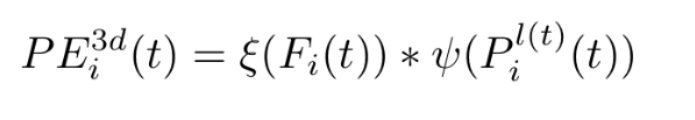where the left side is the 3D position embedding considering image features, ξ(F) is the output of MLP with image features as input to which the Sigmoid function is applied, P is the coordinate value of the grid point on the coordinate system based on the position at time t, ψ is the MLP that transforms it, and the 3D position embedding obtained with ψ is multiplied by a softmask using image features for each element The image is that a softmask is applied to the 3D position embedding obtained by ψ using image features for each element.

PETR v2 performs 3D object detection as in PETR, but in addition it performs segmentation and 3D lane detection on a bird's eye view (BEV) at the same time. These tasks are performed using the same Transformer as for object detection, updating the query and feeding it into the head for each task.

BEV segmentation: First, the bird's-eye view is divided into several segments = patches. In the figure below, a patch consists of 4 2x2 pixels. A point in the patch is considered as an anchor point, and is put into MLP as in object detection, and a query is computed. This query is then input to the same transformer as in object detection, updated, and then put back on the original bird's-eye view, upsampled, and finally the class of each pixel in the bird's-eye view is estimated and learned using focal loss. In our evaluation experiments, we use Driveable area, Lane, and Vehicle as classes, the size of the bird's eye view is 200pixel x 200pixel, and the number of patches (=query count) is 25x25=625.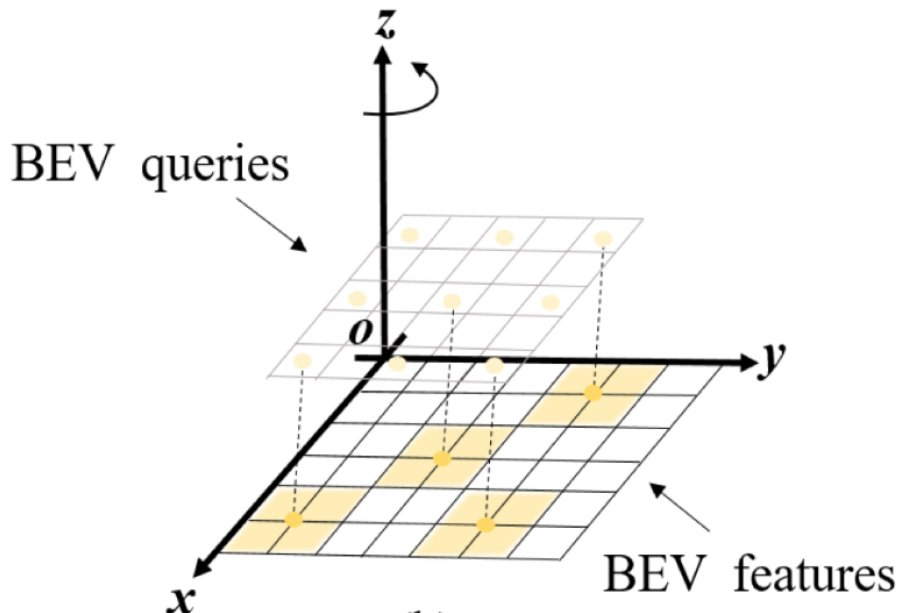3D lane detection: Instead of 3D anchor points used for object detection, an ordered set of anchor points called anchor lane is prepared. This anchor lane is set to be parallel to the y-axis (= direction of vehicle travel) as shown in the figure below.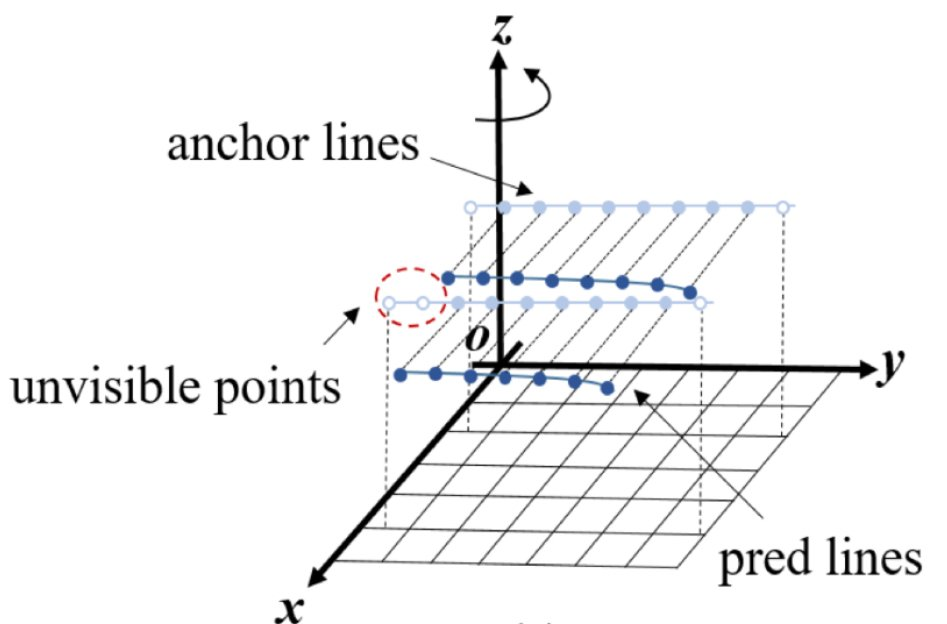Each point of this anchor line is transformed by MLP to compute a query, which is then input to the Transformer, which uses the output to estimate the class of the lane, the x- and z-axis offset from the anchor point (i.e., the y coordinate is and whether the point is visible or not. We use focal loss for the lane class and whether the point is visible, and L1 loss for the offset from the anchor point.

In the evaluation test, the number of points comprising each anchor line is 10, with y-coordinates [5, 10, 15, 20, 30, 40, 50, 60, 80, 100] and the number of queries is 100 (that is, the number of anchor lines is 10).

Given the way the anchor line is set up, it does not seem to be intended to detect lanes in the direction directly opposite to the direction you are traveling, such as at an intersection.

## Evaluation

The 3D object detection and bird's-eye view segmentation tasks are evaluated using the nuscenes dataset, while lane detection is evaluated using the OpenLane dataset.

The table below compares the accuracy of 3D object detection, with a line drawn between PETR and BEVFormer, above which the methods use only one time observation, and below which the methods use multiple time frames of observation. The PETRv2 method performs better with larger input size, and the Backbone method performs better with Res-101 than with Res-50, both of which are reasonable results. outperform PETRv2, but the reason for this is not specifically mentioned in the paper.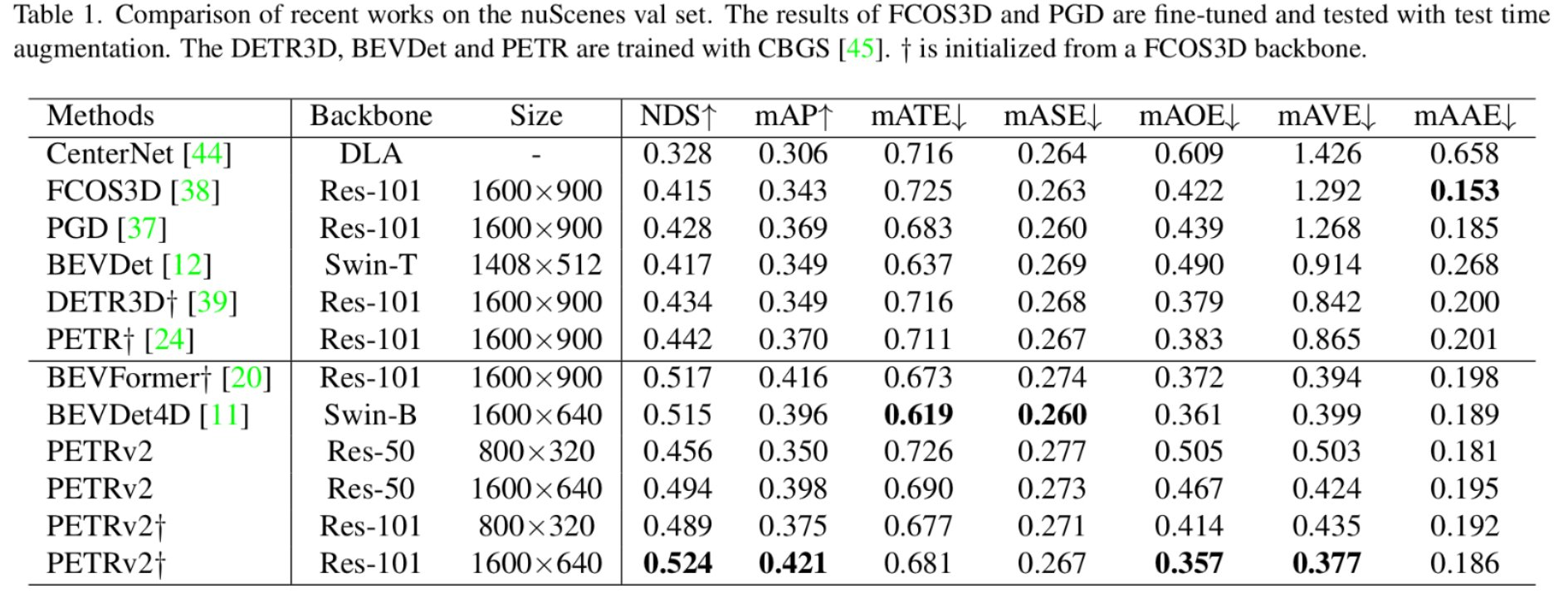The table below shows the results for the bird's eye view Segmentationt, where PETRv2* represents the results when using external training data. Comparing BEVFormer and PETRv2 using the same training data, PETRv2 is better for Lane, but PETRv2 is better for Drive and BEBFormer is better for Vehicle.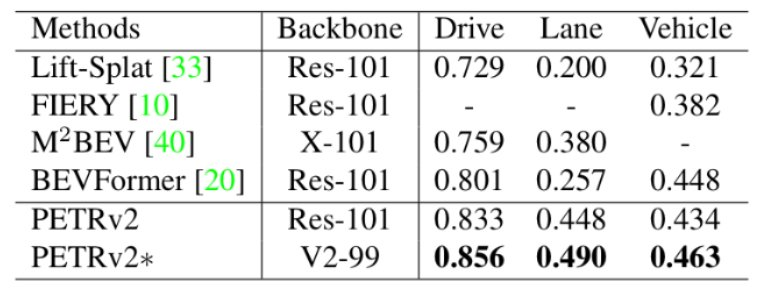The table below shows the results for lane detection, where PETRv2-{V,E} shows the results when VoVNetV2 and EfficientNet are used as backbone and V* shows the results when the number of anchor points is increased from 100 to 400.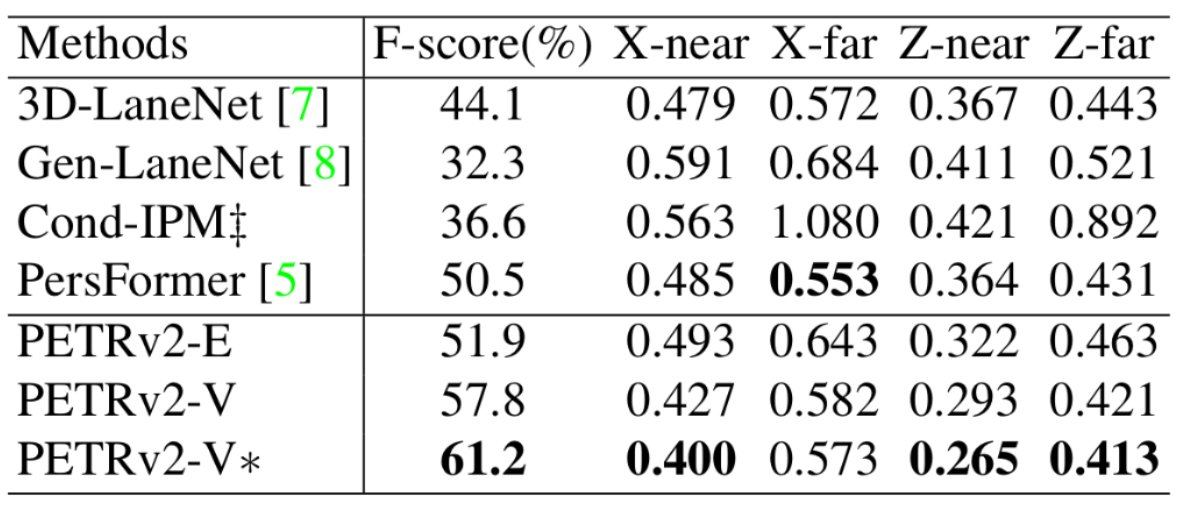Despite the claim of multitask learning, the results of different settings for each task are posted, which is disconcerting, but this is probably because there was no setting that would be SOTA for any task, so the best one was produced for each task.

## Summary

In this article, we introduced a method for 3D object detection, bird's-eye view segmentation, and lane detection using Transformer based on multiple camera images only. This field attracted a lot of attention when Tesla announced its technology in 2021 (the paper has not been published), and is currently evolving rapidly, so we will continue to follow the trends.

If you have any suggestions for improvement of the content of the article,